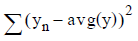# 5.4.5 - Regression Trees - Teradata Warehouse Miner

## Teradata Warehouse Miner User Guide - Volume 3Analytic Functions

Product
Release Number
5.4.5
Published
February 2018
Language
English (United States)
Last Update
2018-05-04
dita:mapPath
yuy1504291362546.ditamap
dita:ditavalPath
ft:empty

Teradata Warehouse Miner provides regression tree models that are built largely on the techniques described in [Breiman, Friedman, Olshen and Stone].

Like classification trees, regression trees utilize SQL in order to extract only the necessary information from the RDBMS instead of extracting all the data from the table. An m x 3 table is returned from the database that has m rows corresponding to the distinct values of an attribute followed by the SUM and SQUARED SUM of the predicted variable and the total number of rows having that attribute value.

Using the formula:the sum of squares for any particular node starting with the root node of all the data is calculated first. The regression tree is built by iteratively splitting nodes and picking the split for that node which will maximize a decrease in the within node sum of squares of the tree. Splitting stops if the minimum number of observations in a node is reached or if all of the predicted variable values are the same.

The value to predict for a leaf node is simply the average of all the predicted values that fall into that leaf during model building.